## 5.397. symmetric_alldifferent_loop

Origin
Constraint

$\mathrm{𝚜𝚢𝚖𝚖𝚎𝚝𝚛𝚒𝚌}_\mathrm{𝚊𝚕𝚕𝚍𝚒𝚏𝚏𝚎𝚛𝚎𝚗𝚝}_\mathrm{𝚕𝚘𝚘𝚙}\left(\mathrm{𝙽𝙾𝙳𝙴𝚂}\right)$

Synonyms

$\mathrm{𝚜𝚢𝚖𝚖𝚎𝚝𝚛𝚒𝚌}_\mathrm{𝚊𝚕𝚕𝚍𝚒𝚏𝚏}_\mathrm{𝚕𝚘𝚘𝚙}$, $\mathrm{𝚜𝚢𝚖𝚖𝚎𝚝𝚛𝚒𝚌}_\mathrm{𝚊𝚕𝚕𝚍𝚒𝚜𝚝𝚒𝚗𝚌𝚝}_\mathrm{𝚕𝚘𝚘𝚙}$, $\mathrm{𝚜𝚢𝚖𝚖}_\mathrm{𝚊𝚕𝚕𝚍𝚒𝚏𝚏𝚎𝚛𝚎𝚗𝚝}_\mathrm{𝚕𝚘𝚘𝚙}$, $\mathrm{𝚜𝚢𝚖𝚖}_\mathrm{𝚊𝚕𝚕𝚍𝚒𝚏𝚏}_\mathrm{𝚕𝚘𝚘𝚙}$, $\mathrm{𝚜𝚢𝚖𝚖}_\mathrm{𝚊𝚕𝚕𝚍𝚒𝚜𝚝𝚒𝚗𝚌𝚝}_\mathrm{𝚕𝚘𝚘𝚙}$.

Argument
 $\mathrm{𝙽𝙾𝙳𝙴𝚂}$ $\mathrm{𝚌𝚘𝚕𝚕𝚎𝚌𝚝𝚒𝚘𝚗}\left(\mathrm{𝚒𝚗𝚍𝚎𝚡}-\mathrm{𝚒𝚗𝚝},\mathrm{𝚜𝚞𝚌𝚌}-\mathrm{𝚍𝚟𝚊𝚛}\right)$
Restrictions
 $\mathrm{𝚛𝚎𝚚𝚞𝚒𝚛𝚎𝚍}$$\left(\mathrm{𝙽𝙾𝙳𝙴𝚂},\left[\mathrm{𝚒𝚗𝚍𝚎𝚡},\mathrm{𝚜𝚞𝚌𝚌}\right]\right)$ $\mathrm{𝙽𝙾𝙳𝙴𝚂}.\mathrm{𝚒𝚗𝚍𝚎𝚡}\ge 1$ $\mathrm{𝙽𝙾𝙳𝙴𝚂}.\mathrm{𝚒𝚗𝚍𝚎𝚡}\le |\mathrm{𝙽𝙾𝙳𝙴𝚂}|$ $\mathrm{𝚍𝚒𝚜𝚝𝚒𝚗𝚌𝚝}$$\left(\mathrm{𝙽𝙾𝙳𝙴𝚂},\mathrm{𝚒𝚗𝚍𝚎𝚡}\right)$ $\mathrm{𝙽𝙾𝙳𝙴𝚂}.\mathrm{𝚜𝚞𝚌𝚌}\ge 1$ $\mathrm{𝙽𝙾𝙳𝙴𝚂}.\mathrm{𝚜𝚞𝚌𝚌}\le |\mathrm{𝙽𝙾𝙳𝙴𝚂}|$
Purpose

All variables associated with the $\mathrm{𝚜𝚞𝚌𝚌}$ attribute of the $\mathrm{𝙽𝙾𝙳𝙴𝚂}$ collection should be pairwise distinct. In addition enforce the following condition: if variable $\mathrm{𝙽𝙾𝙳𝙴𝚂}\left[i\right].\mathrm{𝚜𝚞𝚌𝚌}$ is assigned value $j$ then variable $\mathrm{𝙽𝙾𝙳𝙴𝚂}\left[j\right].\mathrm{𝚜𝚞𝚌𝚌}$ is assigned value $i$. Note that $i$ and $j$ are not necessarily distinct. This can be interpreted as a graph-covering problem where one has to cover a digraph $G$ with circuits of length two or one in such a way that each vertex of $G$ belongs to a single circuit.

Example
$\left(\begin{array}{c}〈\begin{array}{cc}\mathrm{𝚒𝚗𝚍𝚎𝚡}-1\hfill & \mathrm{𝚜𝚞𝚌𝚌}-1,\hfill \\ \mathrm{𝚒𝚗𝚍𝚎𝚡}-2\hfill & \mathrm{𝚜𝚞𝚌𝚌}-4,\hfill \\ \mathrm{𝚒𝚗𝚍𝚎𝚡}-3\hfill & \mathrm{𝚜𝚞𝚌𝚌}-3,\hfill \\ \mathrm{𝚒𝚗𝚍𝚎𝚡}-4\hfill & \mathrm{𝚜𝚞𝚌𝚌}-2\hfill \end{array}〉\hfill \end{array}\right)$

The $\mathrm{𝚜𝚢𝚖𝚖𝚎𝚝𝚛𝚒𝚌}_\mathrm{𝚊𝚕𝚕𝚍𝚒𝚏𝚏𝚎𝚛𝚎𝚗𝚝}_\mathrm{𝚕𝚘𝚘𝚙}$ constraint holds since:

• We have two loops respectively corresponding to $\mathrm{𝙽𝙾𝙳𝙴𝚂}\left[1\right].\mathrm{𝚜𝚞𝚌𝚌}=1$ and $\mathrm{𝙽𝙾𝙳𝙴𝚂}\left[3\right].\mathrm{𝚜𝚞𝚌𝚌}=3$.

• We have one circuit of length 2 corresponding to $\mathrm{𝙽𝙾𝙳𝙴𝚂}\left[2\right].\mathrm{𝚜𝚞𝚌𝚌}=4⇔\mathrm{𝙽𝙾𝙳𝙴𝚂}\left[4\right].\mathrm{𝚜𝚞𝚌𝚌}=2$.

Figure 5.397.1 provides a second example involving a $\mathrm{𝚜𝚢𝚖𝚖𝚎𝚝𝚛𝚒𝚌}_\mathrm{𝚊𝚕𝚕𝚍𝚒𝚏𝚏𝚎𝚛𝚎𝚗𝚝}_\mathrm{𝚕𝚘𝚘𝚙}$ constraint.

##### Figure 5.397.1. (A) Magic square Duerer where cells that belong to a same cycle are coloured identically by a colour different from grey; each cell has an index in its upper left corner (in red) and a value (in blue). (B) Corresponding graph where there is an arc from node $i$ to node $j$ if and only if the value of cell $i$ is equal to the index of cell $j$. (C) Collection of nodes passed to the $\mathrm{𝚜𝚢𝚖𝚖𝚎𝚝𝚛𝚒𝚌}_\mathrm{𝚊𝚕𝚕𝚍𝚒𝚏𝚏𝚎𝚛𝚎𝚗𝚝}_\mathrm{𝚕𝚘𝚘𝚙}$ constraint: the four self-loops of the graph correspond to the four grey cells of the magic square such that the value of the cell (in blue) is equal to the index of the cell (in red).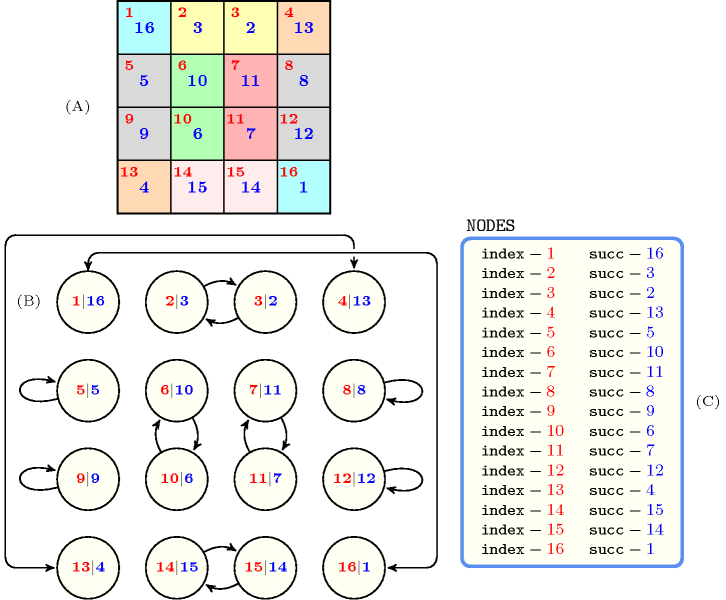All solutions

Figure 5.397.2 gives all solutions to the following non ground instance of the $\mathrm{𝚜𝚢𝚖𝚖𝚎𝚝𝚛𝚒𝚌}_\mathrm{𝚊𝚕𝚕𝚍𝚒𝚏𝚏𝚎𝚛𝚎𝚗𝚝}_\mathrm{𝚕𝚘𝚘𝚙}$ constraint: ${S}_{1}\in \left[2,5\right]$, ${S}_{2}\in \left[1,3\right]$, ${S}_{3}\in \left[1,4\right]$, ${S}_{4}\in \left[2,4\right]$, ${S}_{5}\in \left[1,5\right]$, $\mathrm{𝚜𝚢𝚖𝚖𝚎𝚝𝚛𝚒𝚌}_\mathrm{𝚊𝚕𝚕𝚍𝚒𝚏𝚏𝚎𝚛𝚎𝚗𝚝}_\mathrm{𝚕𝚘𝚘𝚙}$$\left(〈1{S}_{1},2{S}_{2},3{S}_{3},4{S}_{4},5{S}_{5}〉\right)$.

##### Figure 5.397.2. All solutions corresponding to the non ground example of the $\mathrm{𝚜𝚢𝚖𝚖𝚎𝚝𝚛𝚒𝚌}_\mathrm{𝚊𝚕𝚕𝚍𝚒𝚏𝚏𝚎𝚛𝚎𝚗𝚝}_\mathrm{𝚕𝚘𝚘𝚙}$ constraint of the All solutions slot; the $\mathrm{𝚒𝚗𝚍𝚎𝚡}$ attribute is displayed as indices of the $\mathrm{𝚜𝚞𝚌𝚌}$ attribute and self loops are coloured in red.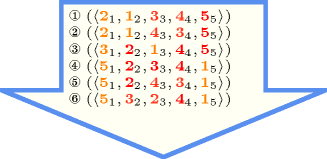Typical
$|\mathrm{𝙽𝙾𝙳𝙴𝚂}|\ge 4$
Symmetry

Items of $\mathrm{𝙽𝙾𝙳𝙴𝚂}$ are permutable.

Algorithm

An arc-consistency filtering algorithm for the $\mathrm{𝚜𝚢𝚖𝚖𝚎𝚝𝚛𝚒𝚌}_\mathrm{𝚊𝚕𝚕𝚍𝚒𝚏𝚏𝚎𝚛𝚎𝚗𝚝}_\mathrm{𝚕𝚘𝚘𝚙}$ constraint is described in [Cymer13], [CymerPhD13]. The algorithm is based on the following ideas:

• First, one can map solutions of the $\mathrm{𝚜𝚢𝚖𝚖𝚎𝚝𝚛𝚒𝚌}_\mathrm{𝚊𝚕𝚕𝚍𝚒𝚏𝚏𝚎𝚛𝚎𝚗𝚝}_\mathrm{𝚕𝚘𝚘𝚙}$ constraint to perfect $\left(g,f\right)$-matchings in a non-bipartite graph derived from the domain of the variables of the constraint where $g\left(x\right)=0$, $f\left(x\right)=1$ for vertices $x$ which have a self-loop, and $g\left(x\right)=f\left(x\right)=1$ for all the remaining vertices. A perfect $\left(g,f\right)$-matching $ℳ$ of a graph is a subset of edges such that every vertex $x$ is incident with the number of edges in $ℳ$ between $g\left(x\right)$ and $f\left(x\right)$.

• Second, Gallai-Edmonds decomposition [Gallai63], [Edmonds65] allows to find out all edges that do not belong any perfect $\left(g,f\right)$-matchings, and therefore prune the corresponding variables.

Counting
 Length ($n$) 2 3 4 5 6 7 8 9 10 Solutions 2 4 10 26 76 232 764 2620 9496

Number of solutions for $\mathrm{𝚜𝚢𝚖𝚖𝚎𝚝𝚛𝚒𝚌}_\mathrm{𝚊𝚕𝚕𝚍𝚒𝚏𝚏𝚎𝚛𝚎𝚗𝚝}_\mathrm{𝚕𝚘𝚘𝚙}$: domains $0..n$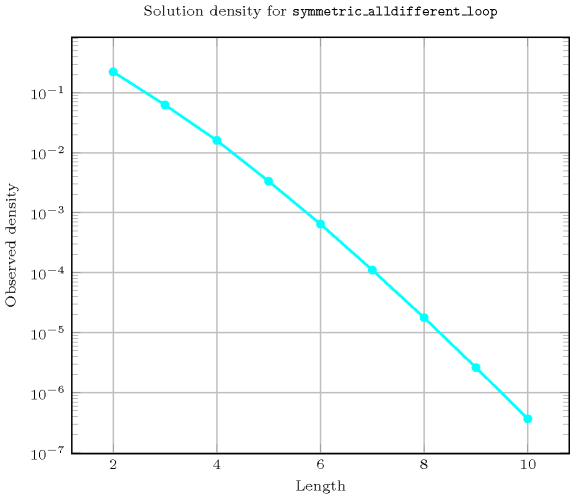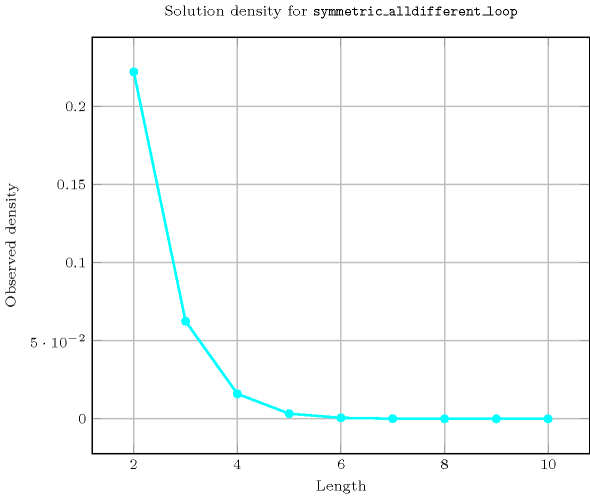Keywords
Cond. implications

$\mathrm{𝚜𝚢𝚖𝚖𝚎𝚝𝚛𝚒𝚌}_\mathrm{𝚊𝚕𝚕𝚍𝚒𝚏𝚏𝚎𝚛𝚎𝚗𝚝}_\mathrm{𝚕𝚘𝚘𝚙}\left(\mathrm{𝙽𝙾𝙳𝙴𝚂}\right)$

implies $\mathrm{𝚙𝚎𝚛𝚖𝚞𝚝𝚊𝚝𝚒𝚘𝚗}$$\left(\mathrm{𝚅𝙰𝚁𝙸𝙰𝙱𝙻𝙴𝚂}:\mathrm{𝙽𝙾𝙳𝙴𝚂}\right)$.

Arc input(s)

$\mathrm{𝙽𝙾𝙳𝙴𝚂}$

Arc generator
$\mathrm{𝐶𝐿𝐼𝑄𝑈𝐸}$$↦\mathrm{𝚌𝚘𝚕𝚕𝚎𝚌𝚝𝚒𝚘𝚗}\left(\mathrm{𝚗𝚘𝚍𝚎𝚜}\mathtt{1},\mathrm{𝚗𝚘𝚍𝚎𝚜}\mathtt{2}\right)$

Arc arity
Arc constraint(s)
 $•\mathrm{𝚗𝚘𝚍𝚎𝚜}\mathtt{1}.\mathrm{𝚜𝚞𝚌𝚌}=\mathrm{𝚗𝚘𝚍𝚎𝚜}\mathtt{2}.\mathrm{𝚒𝚗𝚍𝚎𝚡}$ $•\mathrm{𝚗𝚘𝚍𝚎𝚜}\mathtt{2}.\mathrm{𝚜𝚞𝚌𝚌}=\mathrm{𝚗𝚘𝚍𝚎𝚜}\mathtt{1}.\mathrm{𝚒𝚗𝚍𝚎𝚡}$
Graph property(ies)
$\mathrm{𝐍𝐀𝐑𝐂}$$=|\mathrm{𝙽𝙾𝙳𝙴𝚂}|$

Graph model

In order to express the binary constraint that links two vertices one has to make explicit the identifier of the vertices.

Parts (A) and (B) of Figure 5.397.3 respectively show the initial and final graph associated with the Example slot. Since we use the $\mathrm{𝐍𝐀𝐑𝐂}$ graph property, the arcs of the final graph are stressed in bold.

##### Figure 5.397.3. Initial and final graph of the $\mathrm{𝚜𝚢𝚖𝚖𝚎𝚝𝚛𝚒𝚌}_\mathrm{𝚊𝚕𝚕𝚍𝚒𝚏𝚏𝚎𝚛𝚎𝚗𝚝}_\mathrm{𝚕𝚘𝚘𝚙}$ constraint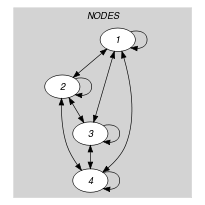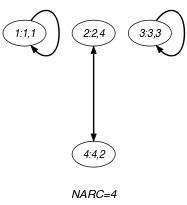(a) (b)
Signature

Since all the $\mathrm{𝚒𝚗𝚍𝚎𝚡}$ attributes of the $\mathrm{𝙽𝙾𝙳𝙴𝚂}$ collection are distinct, and because of the first condition $\mathrm{𝚗𝚘𝚍𝚎𝚜}\mathtt{1}.\mathrm{𝚜𝚞𝚌𝚌}=\mathrm{𝚗𝚘𝚍𝚎𝚜}\mathtt{2}.\mathrm{𝚒𝚗𝚍𝚎𝚡}$ of the arc constraint, each vertex of the final graph has at most one successor. Therefore the maximum number of arcs of the final graph is equal to the maximum number of vertices $|\mathrm{𝙽𝙾𝙳𝙴𝚂}|$ of the final graph. So we can rewrite $\mathrm{𝐍𝐀𝐑𝐂}=|\mathrm{𝙽𝙾𝙳𝙴𝚂}|$ to $\mathrm{𝐍𝐀𝐑𝐂}\ge |\mathrm{𝙽𝙾𝙳𝙴𝚂}|$ and simplify $\underline{\overline{\mathrm{𝐍𝐀𝐑𝐂}}}$ to $\overline{\mathrm{𝐍𝐀𝐑𝐂}}$.